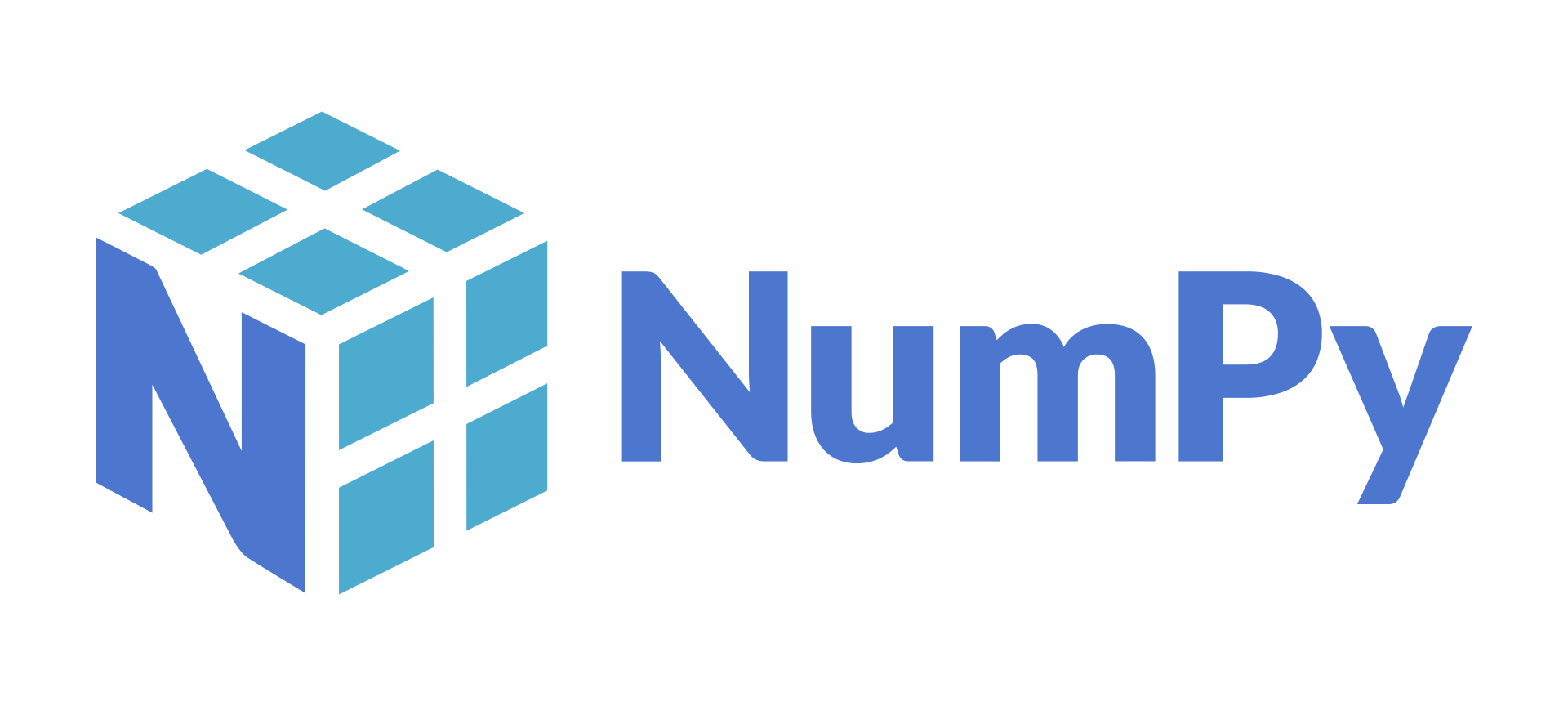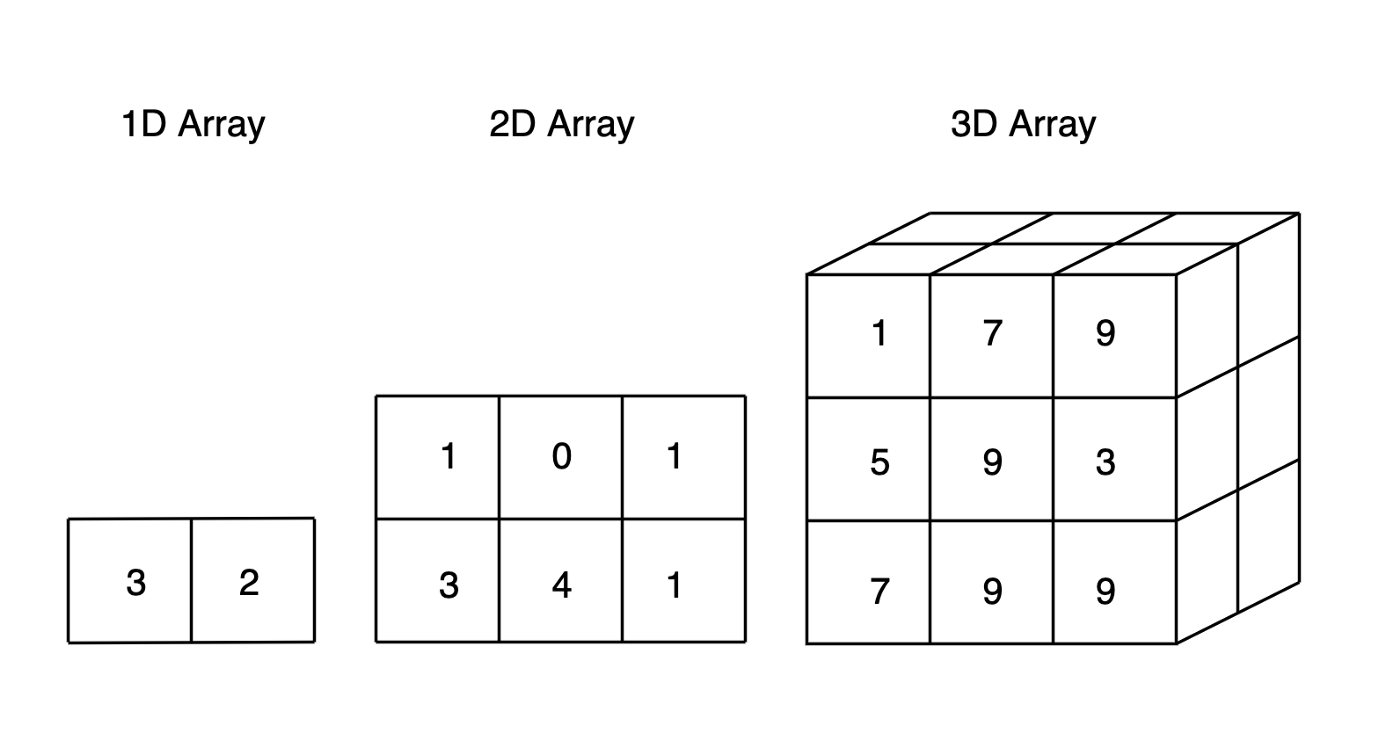# Python Data Processing: What is NumPy?

Learn why you should use NumPy for data processing pipelines.
By Boris Delovski • Updated on Mar 2, 2023Before I start explaining how NumPy functions, I'll explain why you would even want to use NumPy over other options. In this article, I will talk about what NumPy is and why you should start using NumPy in your data processing pipelines.

## What is NumPy?

One of the first things beginners learn when they start programming in Python is that there is usually no need to write your code from scratch. Instead, what programmers do is leverage the power of existing libraries, packages, and modules to solve whatever problem they are working on.

When it comes to general scientific computing and data analysis, NumPy is the premiere choice. Even though Pandas is the package that you use for data analysis and data processing nowadays, it is essentially built on top of NumPy, so it is a good idea to learn NumPy instead of skipping it.

In order to have a good understanding of how Pandas works, you need to understand at the very least the basics of how NumPy works.Image Source: https://en.wikipedia.org/wiki/NumPy

The word NumPy is short for Numerical Python, which by itself could give you an idea about what is NumPy used for. NumPy is a Python package designed for scientific computing and data analysis. It is most often used in fields connected to science and engineering and is the universal standard for working with numbers in Python. NumPy is important because it introduces a new data structure called an array. Typically in Python, you use lists to store data, but in the case of NumPy, you use multidimensional arrays called ndarrays.

In practice, you'll mostly work with multidimensional data, so it is much more practical to store that multidimensional data in arrays than in lists. That doesn't mean that arrays can completely replace lists since there are still situations where using lists is the better option, but in data processing, they are few and far between.

## Why Use NumPy?

I've already mentioned that programmers prefer using arrays over lists for data processing, but let's now explain why.

There are three main reasons why programmers prefer using arrays from the NumPy package over Python lists:

• Optimized Performance
• Ease of use
• Popularity

Article continues below

### Optimizing Performance

When it comes to performance, one of the reasons NumPy offers great performance is because it is based on optimized C, C++, and Fortran code. This means that, even though you are using Python, which is an interpreted language, you can still gain the benefits that come with compiled code.

Another very important reason why NumPy is fast is that the data gets stored in the aforementioned multidimensional arrays. A NumPy ndarray is fundamentally different from a Python list: it stores homogenous data, while Python lists store heterogeneous data.

Homogeneous data structures can only store a single type of data, such as numeric, integer or character.

Heterogeneous data structures can store more than one type of data at the same time.

While a lot of the speed comes from the fact that you're working with homogenous and not heterogeneous data, a part of your performance increase also stems from the difference in memory allocation. Contiguous memory allocation is a method of allocating a single contiguous section of memory to a process or file that needs it.Multidimensional arrays, Image Source: https://towardsdatascience.com

In contrast, non-contiguous memory allocation is a method allocating separate memory sections, which could be in different locations on the hard drive. Arrays use contiguous memory allocation, while lists use non-contiguous memory allocation, which makes arrays much more space-efficient.

In terms of how you process data, by using NumPy arrays, you can avoid using loops when performing linear algebra and standard math operations. Python loops are known to be very inefficient when used for vectorized operations, so the ability to perform such operations without using loops is very useful.

### Ease of Use

NumPy is very easy to use. The API it offers is pretty high level, which makes performing even the most complex operations very straightforward. Even beginner Python programmers can get the hang of using NumPy very quickly and can find a solution to any problem they run into with the help of cheat sheets and a little bit of searching on the Internet.

With NumPy, performing operations such as inspecting arrays, array mathematics, comparing values, sorting, and much more become simple one-liners. You can also perform advanced operations that would be impossible to perform with lists, such as multidimensional slicing (which is also achieved by using a single line of code).

There is also the added benefit of carrying over knowledge to other libraries. Learning the API that NumPy uses is also a good investment in the future because all of the most important data processing Python libraries (Pandas, Scikit-Learn, SciPy, etc.) use it in some way, so if you start learning data processing by learning NumPy you will build a strong foundation for learning everything else you will need in the future.

### Popularity

Alongside Pandas, NumPy is probably the most well-known library for data processing. NumPy is an open-source Python project, distributed under a BSD license. Anybody can use it for any purpose, so it is no surprise that it is so popular. Because of its popularity, NumPy enjoys a lot of community support. If you at any point in time run into a problem, you will probably find a solution to it very quickly on the internet.

This is not only important for beginner programmers, but also for experienced programmers since it further streamlines the learning process and makes learning NumPy a lot easier and less frustrating. It also means that you don't need to become an expert in NumPy before starting to use it in practice, even in production environments. As long as you learn the basics, you can start implementing NumPy into your pipelines to make them faster and more efficient.

## Key Takeaways About the Benefits of NumPy

• NumPy, as an open-source library, enjoys a lot of support as one of the most popular data processing libraries out there.
• In NumPy, you store homogenous data in very space-efficient multidimensional arrays called ndarrays using contiguous memory allocation, which leads to an increase in performance.
• The API of NumPy is the basis of what all other data processing libraries in Python use, so learning NumPy is a great investment in the future.# Boris Delovski

Boris is a data science trainer and consultant who is passionate about sharing his knowledge with others.

Before Edlitera, Boris applied his skills in several industries, including neuroimaging and metallurgy, using data science and deep learning to analyze images.